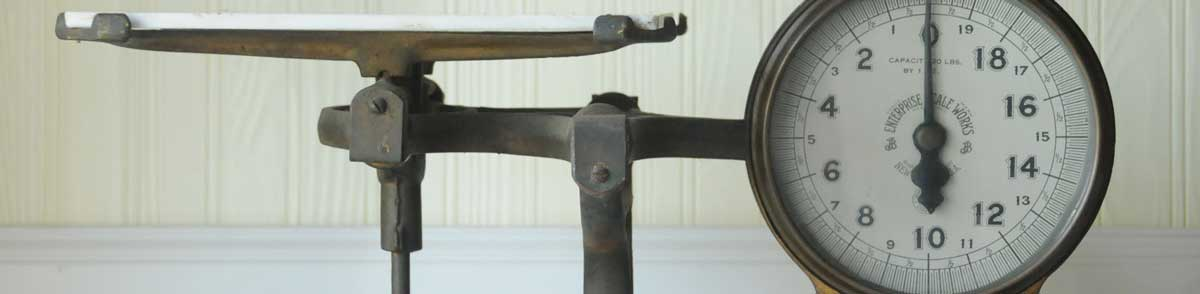# mL to oz Conversion (Milliliters to Ounces)

Enter the volume in milliliters below to calculate the weight in ounces.

Results in Ounces:1 ml = 0.03527396195 oz

## How to Convert Milliliters to OuncesTo convert a milliliter measurement to an ounce measurement, divide the volume multiplied by the density of the ingredient or material by 28.349523.

You can use this simple formula to convert:

ounces = milliliters × ingredient density28.349523

Thus, the volume in ounces is equal to the milliliters times the density of the ingredient or material divided by 28.349523.

For example, here's how to convert 5 milliliters to ounces for an ingredient with a density of 0.7 g/mL.
5 ml = 5 × 0.728.349523 = 0.123458866824 oz

This calculator converts to ounces in weight, if you're trying to convert to a volume measurement in fluid ounces try our milliliters to fluid ounces calculator.

Milliliters and ounces are both commonly used to measure cooking ingredients.

For cooking applications, most chefs suggest measuring dry ingredients by weight rather than volume to improve accuracy in the measurements. The density of dry ingredients can vary for a variety of reasons, such as compaction.

The best way to ensure an accurate conversion is to use a scale. When a scale is not available, a calculator like the one above is a good way to estimate the volume to weight conversion.

## Milliliters

The milliliter is a unit of volume equal to 1 cubic centimeter, 1/1,000 of a liter, or about 0.061 cubic inches.

The milliliter is an SI unit of volume in the metric system. In the metric system, "milli" is the prefix for 10-3. A milliliter is sometimes also referred to as a millilitre. Milliliters can be abbreviated as ml, and are also sometimes abbreviated as mL or mℓ. For example, 1 milliliter can be written as 1 ml, 1 mL, or 1 mℓ.

Milliliters are sometimes expressed using the abbreviation cc for medical dosage, which is the abbreviation for the cubic centimeter.

## Ounces

One ounce is a unit of mass/weight equal to 1/16 of a pound. The common ounce should not be confused with the troy ounce, which is equal to 1/12 of a troy pound.

The ounce is a US customary and imperial unit of weight. Ounces can be abbreviated as oz; for example, 1 ounce can be written as 1 oz.

## Milliliter to Ounce Conversion Table

Milliliter measurements converted to ounces for commonly used ingredients.
Volume in Milliliters: Weight in Ounces of:
Water Milk Cooking Oil All Purpose Flour Granulated Sugar
1 ml 0.035274 oz 0.036685 oz 0.031041 oz 0.01866 oz 0.024692 oz
2 ml 0.070548 oz 0.07337 oz 0.062082 oz 0.03732 oz 0.049384 oz
3 ml 0.105822 oz 0.110055 oz 0.093123 oz 0.05598 oz 0.074075 oz
4 ml 0.141096 oz 0.14674 oz 0.124164 oz 0.07464 oz 0.098767 oz
5 ml 0.17637 oz 0.183425 oz 0.155205 oz 0.0933 oz 0.123459 oz
6 ml 0.211644 oz 0.22011 oz 0.186247 oz 0.11196 oz 0.148151 oz
7 ml 0.246918 oz 0.256794 oz 0.217288 oz 0.130619 oz 0.172842 oz
8 ml 0.282192 oz 0.293479 oz 0.248329 oz 0.149279 oz 0.197534 oz
9 ml 0.317466 oz 0.330164 oz 0.27937 oz 0.167939 oz 0.222226 oz
10 ml 0.35274 oz 0.366849 oz 0.310411 oz 0.186599 oz 0.246918 oz
11 ml 0.388014 oz 0.403534 oz 0.341452 oz 0.205259 oz 0.27161 oz
12 ml 0.423288 oz 0.440219 oz 0.372493 oz 0.223919 oz 0.296301 oz
13 ml 0.458562 oz 0.476904 oz 0.403534 oz 0.242579 oz 0.320993 oz
14 ml 0.493835 oz 0.513589 oz 0.434575 oz 0.261239 oz 0.345685 oz
15 ml 0.529109 oz 0.550274 oz 0.465616 oz 0.279899 oz 0.370377 oz
16 ml 0.564383 oz 0.586959 oz 0.496657 oz 0.298559 oz 0.395068 oz
17 ml 0.599657 oz 0.623644 oz 0.527698 oz 0.317219 oz 0.41976 oz
18 ml 0.634931 oz 0.660329 oz 0.55874 oz 0.335879 oz 0.444452 oz
19 ml 0.670205 oz 0.697013 oz 0.589781 oz 0.354539 oz 0.469144 oz
20 ml 0.705479 oz 0.733698 oz 0.620822 oz 0.373199 oz 0.493835 oz
21 ml 0.740753 oz 0.770383 oz 0.651863 oz 0.391858 oz 0.518527 oz
22 ml 0.776027 oz 0.807068 oz 0.682904 oz 0.410518 oz 0.543219 oz
23 ml 0.811301 oz 0.843753 oz 0.713945 oz 0.429178 oz 0.567911 oz
24 ml 0.846575 oz 0.880438 oz 0.744986 oz 0.447838 oz 0.592603 oz
25 ml 0.881849 oz 0.917123 oz 0.776027 oz 0.466498 oz 0.617294 oz
26 ml 0.917123 oz 0.953808 oz 0.807068 oz 0.485158 oz 0.641986 oz
27 ml 0.952397 oz 0.990493 oz 0.838109 oz 0.503818 oz 0.666678 oz
28 ml 0.987671 oz 1.0272 oz 0.86915 oz 0.522478 oz 0.69137 oz
29 ml 1.0229 oz 1.0639 oz 0.900192 oz 0.541138 oz 0.716061 oz
30 ml 1.0582 oz 1.1005 oz 0.931233 oz 0.559798 oz 0.740753 oz
31 ml 1.0935 oz 1.1372 oz 0.962274 oz 0.578458 oz 0.765445 oz
32 ml 1.1288 oz 1.1739 oz 0.993315 oz 0.597118 oz 0.790137 oz
33 ml 1.164 oz 1.2106 oz 1.0244 oz 0.615778 oz 0.814829 oz
34 ml 1.1993 oz 1.2473 oz 1.0554 oz 0.634437 oz 0.83952 oz
35 ml 1.2346 oz 1.284 oz 1.0864 oz 0.653097 oz 0.864212 oz
36 ml 1.2699 oz 1.3207 oz 1.1175 oz 0.671757 oz 0.888904 oz
37 ml 1.3051 oz 1.3573 oz 1.1485 oz 0.690417 oz 0.913596 oz
38 ml 1.3404 oz 1.394 oz 1.1796 oz 0.709077 oz 0.938287 oz
39 ml 1.3757 oz 1.4307 oz 1.2106 oz 0.727737 oz 0.962979 oz
40 ml 1.411 oz 1.4674 oz 1.2416 oz 0.746397 oz 0.987671 oz

## References

1. National Institute of Standards & Technology, Metric Cooking Resources, https://www.nist.gov/pml/weights-and-measures/metric-cooking-resources
2. National Institute of Standards and Technology, Specifications, Tolerances, and Other Technical Requirements for Weighing and Measuring Devices, Handbook 44 - 2019 Edition, https://nvlpubs.nist.gov/nistpubs/hb/2019/NIST.HB.44-2019.pdf
3. James Bucki, Troy Ounce vs. Ounce, The Spruce, https://www.thesprucecrafts.com/troy-ounce-vs-ounce-4588158